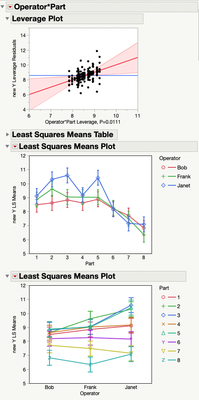Our World Statistics Day conversations have been a great reminder of how much statistics can inform our lives. Do you have an example of how statistics has made a difference in your life? Share your story with the Community!
Choose Language Hide Translation Bar

## LS Means Plots in Fit Model factorial analyses

Currently, the X-axis variable in 2-way factorial is either one factor or the other factor (alternating by using Shift), but it's better to be able to see both alternative plots together (one on top of the other).

### Defect ID: S0835938Staff

This was addressed in JMP 14 and later. To see an example, we can use the sample data file 3 Factors Crossed.jmp:

``````open("\$SAMPLE_DATA\Variability Data\3 Factors Crossed.jmp");
Fit Model(
Y( :new Y ),
Effects(
:Operator,
:Part,
:Operator * :Part,
:Instrument,
:Operator * :Instrument,
:Part * :Instrument,
:Operator * :Part * :Instrument
),
Personality( "Standard Least Squares" ),
Emphasis( "Effect Leverage" ),
Run(
:new Y << {Summary of Fit( 1 ), Analysis of Variance( 1 ),
Parameter Estimates( 1 ), Lack of Fit( 0 ), Scaled Estimates( 0 ),
Plot Actual by Predicted( 1 ), Plot Regression( 0 ),
Plot Residual by Predicted( 1 ), Plot Studentized Residuals( 0 ),
Plot Effect Leverage( 1 ), Plot Residual by Normal Quantiles( 0 ),
Box Cox Y Transformation( 0 ), {:Operator * :Part <<
{Least Squares Means Plot( Overlay Term List( Operator ) ),
Least Squares Means Plot( Overlay Term List( Part ) )}, :Operator * :Part *
:Instrument << {Least Squares Means Plot(
Overlay Term List( Operator ),
Plot By Term List( Part )
)}}}
),
SendToReport(
Dispatch( {"Response new Y"}, "Operator", OutlineBox, {Close( 1 )} ),
Dispatch( {"Response new Y"}, "Part", OutlineBox, {Close( 1 )} ),
Dispatch( {"Response new Y"}, "Instrument", OutlineBox, {Close( 1 )} ),
Dispatch(
{"Response new Y"},
"Operator*Instrument",
OutlineBox,
{Close( 1 )}
),
Dispatch( {"Response new Y"}, "Part*Instrument", OutlineBox, {Close( 1 )} )
)
);``````Community Manager
Status changed to: Delivered